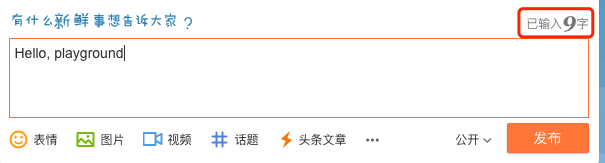## 01 需求分析

• 普通文字
• 标点符号
• 图片
• 链接（包含各种协议的链接）
• 代码

• 空格（包括换行）不算字数；
• HTML 标签需要剔除；
• 编码方式：假定为 UTF-8 编码；
• 标点符号算不算做字数。如果算，像括号这样的按 2 个字算；
• 链接怎么算？一个链接约定为 1 个字可能更合适，大概阅读时只是把它当链接，而不太会关心链接由什么字母组成；
• 图片不算做字数，但如果计算阅读时间，可能需要适当考虑图片的影响；
• 对于技术文章，代码是最麻烦的。统计代码字数感觉是没多大意义的。统计代码行数可能更有意义；

## 02 Go 语言实现

### 纯英文

``````func TotalWords(s string) int {
n := 0
inWord := false
for _, r := range s {
wasInWord := inWord
inWord = !unicode.IsSpace(r)
if inWord && !wasInWord {
n++
}
}
return n
}
``````

``````len(strings.Fields(s))
``````

``````s1 := "Hello,playground"
s2 := "Hello, playground"
``````

### 规范排版### 中英文混合

``````func RuneCountInString(s string) (n int)
``````

``````str := "你好世界"
fmt.Println(utf8.RuneCountInString(str))
``````

``````func TotalWords(s string) int {
wordCount := 0

plainWords := strings.Fields(s)
for _, word := range plainWords {
runeCount := utf8.RuneCountInString(word)
if len(word) == runeCount {
wordCount++
} else {
wordCount += runeCount
}
}

return wordCount
}
``````

``````func TestTotalWords(t *testing.T) {
tests := []struct {
name  string
input string
want  int
}{
{"en1", "hello,playground", 2},
{"en2", "hello, playground", 2},
{"cn1", "你好世界", 4},
{"encn1", "Hello你好世界", 5},
{"encn2", "Hello 你好世界", 5},
}
for _, tt := range tests {
t.Run(tt.name, func(t *testing.T) {
if got := wordscount.TotalWords(tt.input); got != tt.want {
t.Errorf("TotalWords() = %v, want %v", got, tt.want)
}
})
}
}
``````

``````// AutoSpace 自动给中英文之间加上空格
func AutoSpace(str string) string {
out := ""

for _, r := range str {
}

return out
}

func addSpaceAtBoundary(prefix string, nextChar rune) string {
if len(prefix) == 0 {
return string(nextChar)
}

r, size := utf8.DecodeLastRuneInString(prefix)
if isLatin(size) != isLatin(utf8.RuneLen(nextChar)) &&
isAllowSpace(nextChar) && isAllowSpace(r) {
return prefix + " " + string(nextChar)
}

return prefix + string(nextChar)
}

func isLatin(size int) bool {
return size == 1
}

func isAllowSpace(r rune) bool {
return !unicode.IsSpace(r) && !unicode.IsPunct(r)
}
``````

### 处理标点和其他类型

``````type Counter struct {
Total     int // 总字数 = Words + Puncts
Words     int // 只包含字符数
Puncts    int // 标点数
Pics      int // 图片数
CodeLines int // 代码行数
}
``````

``````func (wc *Counter) Stat(str string) {
wc.Pics = len(imgReg.FindAllString(str, -1))

// 剔除 HTML
str = StripHTML(str)

str = AutoSpace(str)

// 普通的链接去除（非 HTML 标签链接）
str = rxStrict.ReplaceAllString(str, " ")
plainWords := strings.Fields(str)

for _, plainWord := range plainWords {
words := strings.FieldsFunc(plainWord, func(r rune) bool {
if unicode.IsPunct(r) {
wc.Puncts++
return true
}
return false
})

for _, word := range words {
runeCount := utf8.RuneCountInString(word)
if len(word) == runeCount {
wc.Words++
} else {
wc.Words += runeCount
}
}
}

wc.Total = wc.Words + wc.Puncts
}

var (
rxStrict = xurls.Strict()
imgReg   = regexp.MustCompile(`<img [^>]*>`)
stripHTMLReplacer = strings.NewReplacer("\n", " ", "</p>", "\n", "<br>", "\n", "<br />", "\n")
)

// StripHTML accepts a string, strips out all HTML tags and returns it.
func StripHTML(s string) string {
// Shortcut strings with no tags in them
if !strings.ContainsAny(s, "<>") {
return s
}
s = stripHTMLReplacer.Replace(s)

// Walk through the string removing all tags
b := GetBuffer()
defer PutBuffer(b)
var inTag, isSpace, wasSpace bool
for _, r := range s {
if !inTag {
isSpace = false
}

switch {
case r == '<':
inTag = true
case r == '>':
inTag = false
case unicode.IsSpace(r):
isSpace = true
fallthrough
default:
if !inTag && (!isSpace || (isSpace && !wasSpace)) {
b.WriteRune(r)
}
}

wasSpace = isSpace

}
return b.String()
}
``````

## 03 总结2584 字

2021-01-14 17:00 +0800